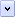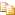Normally, statements in a JScript script are executed one after the other, in the order in which they are written. This is called sequential execution, and is the default direction of program flow.

An alternative to sequential execution transfers the program flow to another part of your script. That is, instead of executing the next statement in the sequence, another statement is executed instead.

To make a script useful, this transfer of control must be done in a logical manner. Transfer of program control is based upon a decision, the result of which is a truth statement (returning a Boolean true or false). You create an expression, then test whether its result is true. There are two main kinds of program structures that accomplish this.

The first is the selection structure. You use it to specify alternate courses of program flow, creating a junction in your program (like a fork in a road). There are four selection structures available in JScript.

• the single-selection structure (if),

• the double-selection structure (if/else),

• the inline ternary operator ?:

• the multiple-selection structure (switch).

The second type of program control structure is the repetition structure. You use it to specify that an action is to be repeated while some condition remains true. When the conditions of the control statement have been met (usually after some specific number of iterations), control passes to the next statement beyond the repetition structure. There are four repetition structures available in JScript.

• the expression is tested at the top of the loop (while),

• the expression is tested at the bottom of the loop (do/while),

• operate on each of an object's properties (for/in).

• counter controlled repetition (for).

You can create quite complex scripts by nesting and stacking selection and repetition control structures.

A third form of structured program flow is provided by exception handling, which is not covered in this document.

# Using Conditional Statements

JScript supports if and if...else conditional statements. In if statements a condition is tested, and if the condition meets the test, the relevant JScript code is executed. In the if...else statement, different code is executed if the condition fails the test. The simplest form of an if statement can be written on one line, but multiline if and if...else statements are much more common.

The following examples demonstrate syntaxes you can use with if and if...else statements. The first example shows the simplest kind of Boolean test. If (and only if) the item between the parentheses evaluates to (or can be coerced to) true, the statement or block of statements after the if is executed.Copy Code
```// The smash() function is defined elsewhere in the code.
// Boolean test of whether newShip is true.
if (newShip)
smash(champagneBottle,bow);

// In this example, the test fails unless both conditions are true.
if (rind.color == "deep yellow " && rind.texture == "large and small wrinkles")
{
theResponse = ("Is it a Crenshaw melon?");
}

// In this example, the test succeeds if either condition is true.
var theReaction = "";
if ((dayOfWeek == "Saturday") || (dayOfWeek == "Sunday"))
{
theReaction = ("I'm off to the beach!");
}
else
{
theReaction = ("Hi ho, hi ho, it's off to work I go!");
}```

# Conditional Operator

JScript also supports an implicit conditional form. It uses a question mark after the condition to be tested (rather than the word if before the condition). It also specifies two alternatives, one to be used if the condition is met and one if it is not. A colon must separate these alternatives.Copy Code
```var hours = "";

// Code specifying that hours contains either the contents of
// theHour, or theHour - 12.

hours += (theHour >= 12) ? " PM" : " AM";```

If you have several conditions to be tested together, and you know that one is more likely to pass or fail than the others, you can use a feature called 'short circuit evaluation' to speed the execution of your script. When JScript evaluates a logical expression, it only evaluates as many sub-expressions as required to get a result.

For example, if you have an AND expression such as ((x == 123) && (y == 42)), JScript first checks if x is 123. If it is not, the entire expression cannot be true, even if y is equal to 42. Hence, the test for y is never made, and JScript returns the value false.

Similarly, if only one of several conditions must be true (using the || operator), testing stops as soon as any one condition passes the test. This is effective if the conditions to be tested involve the execution of function calls or other complex expressions. With this in mind, when you write Or expressions, place the conditions most likely to be true first. When you write And expressions, place the conditions most likely to be false first.

A benefit of designing your script in this manner is that runsecond() will not be executed in the following example if runfirst() returns 0 or false.Copy Code
```if ((runfirst() == 0) || (runsecond() == 0)) {
// some code
}```

# Using Loops

There are several ways to execute a statement or block of statements repeatedly. In general, repetitive execution is called looping or iteration. An iteration is simply a single execution of a loop. It is typically controlled by a test of a variable, where the value of which is changed each time the loop is executed. JScript supports four types of loops: for loops, for...in loops, while loops, do...while loops.

# Using for Loops

The for statement specifies a counter variable, a test condition, and an action that updates the counter. Before each iteration of the loop, the condition is tested. If the test is successful, the code inside the loop is executed. If the test is unsuccessful, the code inside the loop is not executed, and the program continues on the first line of code immediately following the loop. After the loop is executed, the counter variable is updated before the next iteration begins.

If the condition for looping is never met, the loop is never executed. If the test condition is always met, an infinite loop results. While the former may be desirable in certain cases, the latter rarely is, so be cautious when writing your loop conditions.Copy Code
```/*
The update expression ("icount++" in the following examples)
is executed at the end of the loop, after the block of statements that forms the
body of the loop is executed, and before the condition is tested.
*/

var howFar = 10; // Sets a limit of 10 on the loop.

var sum = new Array(howFar); // Creates an array called sum with 10 members, 0 through 9.
var theSum = 0;
sum = 0;

for(var icount = 0; icount < howFar; icount++) { // Counts from 0 through 9 in this case.
theSum += icount;
sum[icount] = theSum;
}

var newSum = 0;
for(var icount = 0; icount > howFar; icount++) { // This isn't executed at all, since icount is not greater than howFar
newSum += icount;
}

var sum = 0;
for(var icount = 0; icount >= 0; icount++) { // This is an infinite loop.
sum += icount;
}```

# Using for...in Loops

JScript provides a special kind of loop for stepping through all the user-defined properties of an object, or all the elements of an array. The loop counter in a for...in loop is a string, not a number. It contains the name of current property or the index of the current array element.

The following code sample should be run from within Internet Explorer, since it uses the alert method, which is not a part of JScript.Copy Code
```// Create an object with some properties
var myObject = new Object();
myObject.name = "James";
myObject.age = "22";
myObject.phone = "555 1234";

// Enumerate (loop through)_all the properties in the object
for (prop in myObject)
{
// This displays "The property 'name' is James", etc.
window.alert("The property '" + prop + "' is " + myObject[prop]);
}```

Although for...in loops look similar to VBScript's For Each...Next loops, they do not work the same way. The JScript for...in loop iterates over properties of JScript objects. The VBScript For Each...Next loop iterates over items in a collection. To loop over collections in JScript, you need to use the Enumerator object. Although some objects, such as those in Internet Explorer, support both VBScript's For Each...Next and JScript's for...in loops, most objects do not.

# Using while Loops

A while loop is similar to a for loop. The difference is, a while loop does not have a built-in counter variable or update expression. If you want to control repetitive execution of a statement or block of statements, but need a more complex rule than simply "run this code n times", use a while loop. The following example uses the Internet Explorer object model and a while loop to ask the user a simple question.Copy Code
```var x = 0;
while ((x != 42) && (x != null))
{
x = window.prompt("What is my favourite number?", x);
}

if (x == null)
elseNote

Because while loops do not have explicit built-in counter variables, they are more vulnerable to infinite looping than the other types of loops. Moreover, because it is not necessarily easy to discover where or when the loop condition is updated, it is easy to write a while loop in which the condition never gets updated. For this reason, you should be careful when you design while loops.

As noted above, there is also a do...while loop in JScript that is similar to the while loop, except that it is guaranteed to always execute at least once, since the condition is tested at the end of the loop, rather than at the start. For example, the loop above can be re-written as:Copy Code
```var x = 0;
do
{
x = window.prompt("What is my favourite number?", x);
} while ((x != 42) && (x != null));

if (x == null)
else

# Using break and continue Statements

In Microsoft JScript, the break statement is used to stop the execution of a loop, if some condition is met. (Note that break is also used to exit a switch block). The continue statement can be used to jump immediately to the next iteration, skipping the rest of the code block, while updating the counter variable if the loop is a for or for...in loop.

The following example builds on the previous example to use the break and continue statements to control the loop.Copy Code
```var x = 0;
do
{
x = window.prompt("What is my favourite number?", x);

// Did the user cancel? If so, break out of the loop
if (x == null)
break;

// Did they enter a number?
// If so, no need to ask them to enter a number.
if (Number(x) == x)
continue;

// Ask user to only enter in numbers

} while (x != 42)

if (x == null)